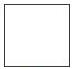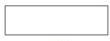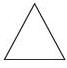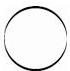Courses

# Which of the following is a square?a)b)c)d)Correct answer is option 'A'. Can you explain this answer? Related Test: Test: Shapes And Space - 3

## Class 1 Question

By Ayansh Songara · Jun 12, 2020 ·Class 1
Ayansh Songara answered Jun 15, 2020
A

Sara Khan answered Jun 20, 2020

Vishal Dhodare answered Sep 02, 2020
A)

Yashina Kapoor answered Jun 12, 2020
(A)this is a square

Bernard Chirwa answered Jun 21, 2020

Amirul Nisha answered Jul 16, 2020
A is a square

Riddhima Manna answered Jul 16, 2020
All sides are equal

Raj Mohd answered Jul 20, 2020
Option a

Square

Anjali Saxena answered Jul 29, 2020
A is the correct answer as it has four equal sides and two equal diagonals

Mahesh Shirsale answered 4 weeks ago
Because it has 4 corners

Deep Tomar answered Aug 28, 2020
F

Daya Kar answered Jul 12, 2020
AA

Peter Sajan answered Jul 18, 2020
A correct

Pooja Telang answered Jul 02, 2020
A is correct

Suman Chauhan answered Aug 01, 2020
Square has four equal sides

Anjali Kumari answered Jul 13, 2020
Because a square have 4 side , so the correct answer is A

Ishu Saini answered Jul 24, 2020
A part

A.

A..

Sudipta Manna answered Sep 07, 2020
Options

Anshu Tiwari answered Aug 23, 2020
C

Divya Verma answered 3 weeks ago
Option (A)

Mohit Kamble answered 2 weeks ago
Square is four side equal that is square

Saphauddin Kuraishi answered 3 weeks ago
Warg

Amrit Aggarwal answered Jun 15, 2020
Option A is correct

Rajani Kamlekar answered Jul 12, 2020

Shyam Agarwal answered Jul 14, 2020
A square has 4 equal sides.

Pallavi Bothra answered Jul 29, 2020
This is square

Ponnala Praveena answered Sep 20, 2020
Opinion A

Anju Dhiman answered Sep 09, 2020
Because the square has four sides

Harpreet Singh answered 3 weeks ago
A is the correct answer because A is a square shape

Arnab Barui answered Sep 02, 2020
Square always has 4 equal sides

Jaya Agrawal answered Sep 02, 2020
A

Nashra Fatma answered Sep 14, 2020
A) all sides is equal is square

Yes

Naveena Noonavath answered Jun 15, 2020
A square is a regular quadrilateral ,has four equal sides and four equal angles and all angles are 90

Narender Sharma answered Jul 03, 2020
,

Tanmayee Guduru answered Jul 16, 2020
A is the answer. because four equal sides

Ankita Dabral answered Sep 02, 2020
The answer of this question is A because square has a 4 sides

Devansh Tiwari 6c answered Aug 17, 2020
Because it has four vertex and four arms and all sides are same

Jalal Khan answered Aug 17, 2020
A is right.
because of equal sides and having 90 angle

Agrim Bansal answered Aug 01, 2020

Gayatri Kumari answered Jul 25, 2020

Kanishka Kumari answered Sep 17, 2020
*The right answer is Option A...*

Palak Singh answered Jul 12, 2020
Square is the a one

Nousin Zaman answered Jul 18, 2020

because square have 4 equal side.

Sanjaydas Bairagi answered Jul 24, 2020
A is a right answer because all the sides are equal

Amritpal Kaur answered Jul 25, 2020
It has 4 vertices

Upendra Solanki answered Jul 26, 2020
Square has 4 equal side

I don't know

Toshani Chaudhary answered Jun 16, 2020
A is square

Shriya Sawant answered Jul 22, 2020

Aijaz Hassan answered Jul 31, 2020
(a). part

Akshita Shukla answered Aug 23, 2020
A because it has four sides and same size of lines

Johan Joseph answered Aug 15, 2020
A is the correct answer which is square it is because a square has equal 4 sides

Sanya Jaiswal answered Sep 17, 2020
Option 'A' Square is because there are 4 option but square is its special features such as square has 4 equal sides

Vijay Singh answered Aug 06, 2020
Option A is square . it's not just like other three option.

Ram Achhaibar Mishra answered Jun 16, 2020

Sunita Prajapati answered Sep 07, 2020
Ans.a

! A;

Nanda Nandini answered Jul 13, 2020
Four sides is correct but in square four sides are equal

Rukmini Govindaraj answered Jul 06, 2020
1st